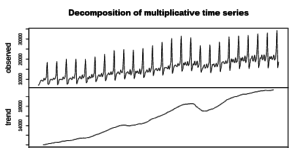Want to share your content on R-bloggers? click here if you have a blog, or here if you don't.R provides powerful tools for forecasting time series data such as sales volumes, population sizes, and earthquake frequencies. A number of those tools are also simple enough to be used without mastering sophisticated underlying theories. This set of exercises is the first in a series offering a possibility to practice in the use of such tools, which include the ARIMA model, exponential smoothing models, and others.
The first set provides a training in exploration of regularly spaced time series data (such as weekly, monthly, and quarterly), which may be useful for selection of a predictive model. The set covers:
– visual inspection of time series,
– estimation of trend and seasonal patterns,
– finding whether a series is stationary (i.e. whether it has a constant mean and variance),
– examination of correlation between lagged values of a time series (autocorrelation).
The exercises make use of functions from the packages `forecast`, and `tseries`. Exercises are based on a dataset on retail sales volume by US clothing and clothing accessory stores for 1992-2016 retrieved from FRED, the Federal Reserve Bank of St. Louis database (download here). The data represent monthly sales in millions of dollars.
For other parts of the series follow the tag forecasting
Answers to the exercises are available here

Exercise 1
Read the data from the file `sales.csv`.

Exercise 2
Transform the data into a time series object of the `ts` type (indicate that the data is monthly, and the starting period is January 1992).
Print the data.

Exercise 3
Plot the time series. Ensure that the `y` axis starts from zero.

Exercise 4
Use the `gghistogram` function from the `forecast` package to visually inspect the distribution of time series values. Add a kernel density estimate and a normal density function to the plot.

Exercise 5
Use the `decompose` function to break the series into seasonal, trend, and irregular components (apply multiplicative decomposition).
Plot the decomposed series.

Exercise 6
Explore the structure of the decomposed object, and find seasonal coefficients (multiples). Identify the three months with the greatest coefficients, and the three months with the smallest coefficients. (Note that the coefficients are equal in different years).

Exercise 7
Check whether the time series is trend-stationary (i.e. its mean and variance are constant with respect to a trend) using the function `kpss.test` from the `tseries` package. (Note that the null hypothesis of the test is that the series is trend-stationary).

Exercise 8
Use the `diff` function to create a differenced time series (i.e. a series that includes differences between the values of the original series), and test it for trend stationarity.

Exercise 9
Plot the differenced time series.

Exercise 10
Use the `Acf` and `Pacf` functions from the `forecast` package to explore autocorrelation of the differenced series. Find at which lags correlation between lagged values is statistically significant at 5% level.